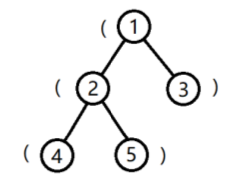# #489. 【CSP-S 2019】括号树

1. () 是合法括号串。
2. 如果 A 是合法括号串,则 (A) 是合法括号串。
3. 如果 A,B 是合法括号串,则 AB 是合法括号串。

1. 字符串 $S$ 的子串是 $S$ 中连续的任意个字符组成的字符串。$S$ 的子串可用起始位置 $l$ 与终止位置 $r$ 来表示,记为 $S(l, r)$ ($1 \leq l \leq r \leq |S |$,$|S |$ 表示 $S$ 的长度)。
2. $S$ 的两个子串视作不同当且仅当它们在 $S$ 中的位置不同，即 $l$ 不同或 $r$ 不同。

$$(1 \times k_1)\ xor\ (2 \times k_2)\ xor\ \cdots \ xor\ (n \times k_n)$$

### 样例1

#### input

5
(()()
1 1 2 2

#### output

6

#### explanation### 限制与约定

$1 \sim 2$$8$$f_i=i-1$
$3 \sim 4$$200 5 \sim 7$$2000$
$8 \sim 10$
$11 \sim 14$$10^5$$f_i=i-1$
$15 \sim 16$
$17 \sim 20$$5 \times 10^5$